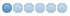# need help in classicladder comparison object

• Coordinates
• Offline
• New Member
•20 Dec 2021 17:50 #229566
i need help how to use exponent and modulus in classicladder  there is not enough examples in linuxcnc documents i want to use them for tool turret  logic  Thanks.

For arithmetic comparison. Is variable %XXX = to this number (or evaluated number)The compare block will be true when comparison is true. you can use most math symbols:
• +, - ,* , /, = (standard math symbols)
• < (less than), > (greater than), <= (less or equal), >= (greater or equal), <> (not equal)
• (, ) grouping
• ^ (exponent),% (modulus),& (and),| (or),. -
• ABS (absolute), MOY (French for average) ,AVG (average)
For example ABS(%W2)=1, MOY(%W1,%W2)<3.No spaces are allowed in the comparison equation. For example %C0.V>%C0.P is a valid comparison expression while %C0.V > %CO.P is not a valid expression.There is a list of Variables down the page that can be used for reading from and writing to ladder objects. When a new compare block is opened be sure and delete the # symbol when you enter a compare.

`%QW0=%IW0%12`